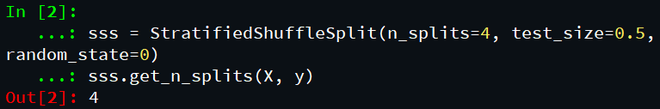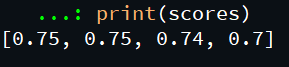Open in App
Not now

# Sklearn.StratifiedShuffleSplit() function in Python

• Last Updated : 10 Oct, 2022

In this article, we’ll learn about the StratifiedShuffleSplit cross validator from sklearn library which gives train-test indices to split the data into train-test sets.

## What is StratifiedShuffleSplit?

StratifiedShuffleSplit is a combination of both ShuffleSplit and StratifiedKFold. Using StratifiedShuffleSplit the proportion of distribution of class labels is almost even between train and test dataset. The major difference between StratifiedShuffleSplit and StratifiedKFold (shuffle=True) is that in StratifiedKFold, the dataset is shuffled only once in the beginning and then split into the specified number of folds. This discards any chances of overlapping of the train-test sets.
However, in StratifiedShuffleSplit the data is shuffled each time before the split is done and this is why there’s a greater chance that overlapping might be possible between train-test sets.

Syntax: sklearn.model_selection.StratifiedShuffleSplit(n_splits=10, *, test_size=None, train_size=None, random_state=None)

Parameters:

n_splits: int, default=10

Number of re-shuffling & splitting iterations.

test_size: float or int, default=None

If float, should be between 0.0 and 1.0 and represent the proportion of the dataset to include in the test split.

train_size: float or int, default=None

If float, should be between 0.0 and 1.0 and represent the proportion of the dataset to include in the train split.

random_state: int

Controls the randomness of the training and testing indices produced.

Below is the Implementation.

Step 1) Import required modules.

## Python3

 `# import the libraries``import` `pandas as pd``from` `sklearn.ensemble ``import` `RandomForestClassifier``from` `sklearn ``import` `preprocessing``from` `sklearn.metrics ``import` `accuracy_score``from` `sklearn.model_selection ``import` `StratifiedShuffleSplit`

Step 2) Load the dataset and identify the dependent and independent variables.

## Python3

 `# convert data set into dataframe``churn_df ``=` `pd.read_csv(r``"ChurnData.csv"``)` `# assign dependent and independent variables``X ``=` `churn_df[[``'tenure'``, ``'age'``, ``'address'``, ``'income'``,``              ``'ed'``, ``'employ'``, ``'equip'``,   ``'callcard'``, ``'wireless'``]]` `y ``=` `churn_df[``'churn'``].astype(``'int'``)`

Step 3) Pre-process data.

## Python3

 `# data pre-processing``X ``=` `preprocessing.StandardScaler().fit(X).transform(X)`

Step 4) Create object of StratifiedShuffleSplit Class.

## Python3

 `# use StratifiedShuffleSplit()``sss ``=` `StratifiedShuffleSplit(n_splits``=``4``, test_size``=``0.5``,``                             ``random_state``=``0``)``sss.get_n_splits(X, y)`

Output:Step 5) Call the instance and split the data frame into training sample and testing sample. The split() function returns indices for the train-test samples. Use a regression algorithm and compare accuracy for each predicted value.

## Python3

 `scores ``=` `[]` `# using regression to get predicted data``rf ``=` `RandomForestClassifier(n_estimators``=``40``, max_depth``=``7``)``for` `train_index, test_index ``in` `sss.split(X, y):``    ``X_train, X_test ``=` `X[train_index], X[test_index]``    ``y_train, y_test ``=` `y[train_index], y[test_index]``    ``rf.fit(X_train, y_train)``    ``pred ``=` `rf.predict(X_test)``    ``scores.append(accuracy_score(y_test, pred))` `# get accuracy of each prediction``print``(scores)`

Output:My Personal Notes arrow_drop_up